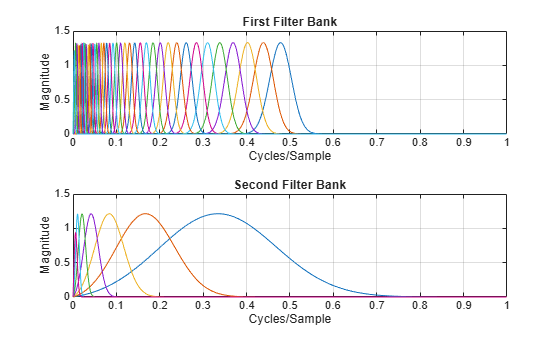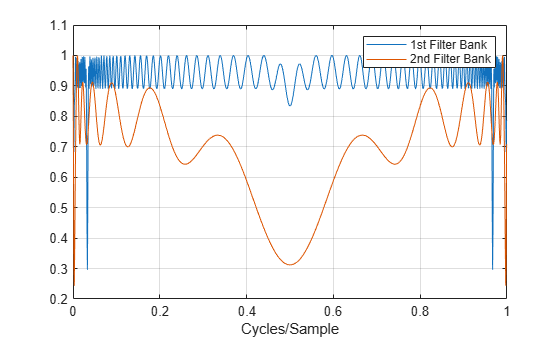Documentation

waveletScattering

Wavelet time scattering

Description

Use the waveletScattering object to create a framework for a wavelet time scattering decomposition using the Gabor (analytic Morlet) wavelet. The framework uses wavelets and a lowpass scaling function to generate low-variance representations of real-valued time series data. Wavelet time scattering yields representations insensitive to translations in the input signal without sacrificing class discriminability. You can use the representations as inputs to a classifier. You can specify the duration of translation invariance and the number of wavelet filters per octave.

Creation

Description

example

sf = waveletScattering creates a framework for a wavelet time scattering decomposition with two filter banks. The first filter bank has a quality factor of eight wavelets per octave. The second filter bank has a quality factor of one wavelet per octave. By default, waveletScattering assumes a signal input length of 1024 samples. The scale invariance length is 512 samples. By default, waveletScattering uses periodic boundary conditions.

example

sf = waveletScattering(Name,Value) creates a framework for wavelet scattering, sf, with properties specified by one or more Name,Value pair arguments. Properties can be specified in any order as Name1,Value1,...,NameN,ValueN. Enclose each property name in quotes.

Note

With the exception of OversamplingFactor, after creation you cannot change a property value of an existing scattering framework. For example, if you have a framework sf with a SignalLength of 2000, you must create a second framework sf2 for a signal with 2001 samples. You cannot assign a different SignalLength to sf.

Properties

expand all

Signal length, specified as a positive integer ≥ 16.

Data Types: double

Sampling frequency in hertz, specified as a positive scalar. If unspecified, frequencies are in cycles/sample and the Nyquist frequency is ½.

Data Types: double

Scattering transform invariance scale, specified as a positive scalar. InvarianceScale specifies the translation invariance of the scattering transform. If you do not specify SamplingFrequency, InvarianceScale is measured in samples. If you specify SamplingFrequency, InvarianceScale is measured in seconds. By default, InvarianceScale is one-half the SignalLength in samples.

InvarianceScale cannot exceed SignalLength in samples.

Example: sf = waveletScattering('SignalLength',1000,'SamplingFrequency',200,'InvarianceScale',5) has the largest possible InvarianceScale.

Data Types: double

Scattering filter bank Q factors, specified as a positive integer or a vector of positive integers. A filter bank Q factor is the number of wavelet filters per octave. Quality factors cannot exceed 32 and must be greater than or equal to 1.

If QualityFactors is specified as a vector, the elements of QualityFactors must be strictly decreasing.

Example: sf = waveletScattering('QualityFactors',[8 2 1]) creates a wavelet scattering framework with three filter banks.

Data Types: double

Signal extension method to apply at the boundary:

• 'periodic' — Extend signal periodically to length 2^ceil(log2(N)), where N is the signal length.

• 'reflection' — Extend signal by reflection to length 2^ceil(log2(2 N)), where N is the signal length.

The signal is extended to match the length of the wavelet filters. The length is always power of two.

The signal extension method is for internal operations. Results are downsampled back onto the scale of the original signal before being returned.

Precision of scattering decomposition:

• 'double' — Double precision

• 'single' — Single precision

Note

• All calculations involving the wavelet scattering framework are carried out in Precision. Wavelet scattering functions such as featureMatrix and filterbank return outputs such as filters in Precision.

• The precision of the output of the scatteringTransform function does not exceed the precision of sf.

Oversampling factor, specified as a nonnegative integer or Inf. The factor specifies how much the scattering coefficients are oversampled with respect to the critically downsampled values. The factor is on a log2 scale. By default, OversamplingFactor is set to 0, which corresponds to critically downsampling the coefficients. You can use numCoefficients to determine the number of coefficients obtained for a scattering framework. To obtain a fully undecimated scattering transform, set OversamplingFactor to Inf.

Setting OversamplingFactor to a value that would result in more coefficients than samples is equivalent to setting OversmplingFactor to Inf. Increasing the OversamplingFactor significantly increases the computational complexity and memory requirements of the scattering transform.

Example: If sf = waveletScattering('OversamplingFactor',2), the scattering transform returns 22 times as many coefficients for each scattering path with respect to the critically sampled number.

Object Functions

 scatteringTransform Wavelet 1-D scattering transform featureMatrix Scattering feature matrix log Natural logarithm of scattering transform filterbank Wavelet time scattering filter banks littlewoodPaleySum Littlewood-Paley sum scattergram Visualize scattering or scalogram coefficients centerFrequencies Wavelet scattering bandpass center frequencies numorders Number of scattering orders numfilterbanks Number of scattering filter banks numCoefficients Number of wavelet scattering coefficients

Examples

collapse all

Create a scattering framework with default values.

sf = waveletScattering
sf =
waveletScattering with properties:

SignalLength: 1024
InvarianceScale: 512
QualityFactors: [8 1]
Boundary: 'periodic'
SamplingFrequency: 1
Precision: 'double'
OversamplingFactor: 0

Plot the wavelet filters used in the first and second filter banks.

[filters,f] = filterbank(sf);
plot(f,filters{2}.psift)
title('First Filter Bank')
xlabel('Cycles/Sample')
ylabel('Magnitude')
grid onfigure
plot(f,filters{3}.psift)
title('Second Filter Bank')
xlabel('Cycles/Sample')
ylabel('Magnitude')
grid onPlot the Littlewood-Paley sums of the filter banks.

[lpsum,f] = littlewoodPaleySum(sf);
figure
plot(f,lpsum)
legend('1st Filter Bank','2nd Filter Bank')
xlabel('Cycles/Sample')
grid onThis example shows how to create and apply a scattering framework with three filter banks to data.

Load in a data set. Create a framework with three filter banks that can be applied to the data.

disp(['Data Sampling Frequency: ',num2str(Fs),' Hz'])
Data Sampling Frequency: 8192 Hz
sf = waveletScattering('SignalLength',numel(y),...
'SamplingFrequency',Fs,...
'QualityFactors',[4 2 1])
sf =
waveletScattering with properties:

SignalLength: 73113
InvarianceScale: 4.4625
QualityFactors: [4 2 1]
Boundary: "periodic"
SamplingFrequency: 8192
Precision: "double"
OversamplingFactor: 0

Inspect the framework. Plot the wavelet filters used in the third filter bank.

[filters,f] = filterbank(sf);
plot(f,filters{4}.psift)
title('Third Filter Bank')
xlabel('Hertz')
ylabel('Magnitude')
grid onPlot the Littlewood-Paley sums of the three filter banks.

[lpsum,f] = littlewoodPaleySum(sf);
figure
plot(f,lpsum)
xlabel('Hertz')
grid on
legend('1st Filter Bank','2nd Filter Bank','3rd Filter Bank')Calculate the wavelet 1-D scattering transform of the data for sf. Visualize the scattergram of the scalogram coefficients for the first filter bank.

[S,U] = scatteringTransform(sf,y);
figure
scattergram(sf,U,'FilterBank',1)Compatibility Considerations

expand all

Not recommended starting in R2019a

 Andén, J., and S. Mallat. "Deep Scattering Spectrum." IEEE Transactions on Signal Processing. Vol. 62, Number 16, 2014, pp. 4114–4128.

 Mallat, S. "Group Invariant Scattering." Communications in Pure and Applied Mathematics. Vol. 65, Number 10, 2012, pp. 1331–1398.

Wavelet Toolbox DocumentationGet trial now